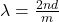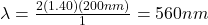What is the longest wavelength λ of light that will exhibit constructive interference at normal incidence? the keratin layers are thin enoug

Question

What is the longest wavelength λ of light that will exhibit constructive interference at normal incidence? the keratin layers are thin enough that you can think of them as representing the surfaces of a 200-nm “film” of air?

in progress 0
6 months 2021-07-26T08:24:17+00:00 1 Answers 6 views 0

560nm

Explanation:

You can use the following expression for thin films:where n is the index of refraction, d is the thicknes of the thin film and m is the order of the diffraction pattern. For keratin layers we can use n=1.40. For the longest wavelenth we use m =1.

Hence, we obtain:HOPE THIS HELPS!!